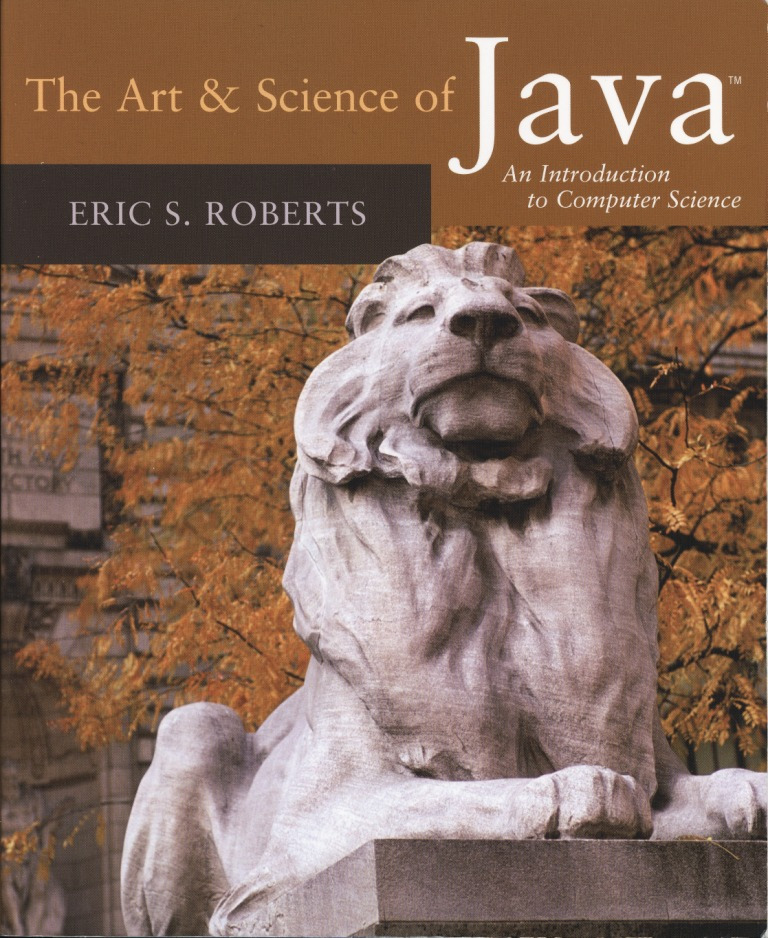# Here are links to example Java programs.

## JAVA PROGRAM EXAMPLES

### Programming exercises from various chapters.Chapter 4 - Problem 5: Calculate Test Score Average

Chapter 4 - Problem 15: Moving Square

Chapter 4 - Problem 15 modified: Bouncing Ball

Chapter 4 - Problem 16: Scrolling Marquis

Chapter 5 - Problem 1: Solve the Quadratic Equation

Chapter 5 - Problem 11: Test to see if Prime Number

Class Assignment 2 - Problem 2: Target Logo

Class Assignment 2 - Problem 4: Pythagorean Theorem

Chapter 9 - Problem 14: Slot Machine

Chapter 8 - Problem 5: SCRABBLE Points

Chapter 8 - Problems 6 & 7: Palindrome word or sentence

Chapter 8 - Problem 8: Make your singular word plural

Assignment 4 - Parts 1, 2, and modified 3 for the web: HANGMAN game!

Assignment 5 - YAHTZEE!

Chapter 10 - Problem 1: Names of Colors

Chapter 10 - Problem 2: Mouse coordinates

Chapter 10 - Problem 3: Watching eyes

Assignment 7: Face Pamphlet (a simple version of Face Book)